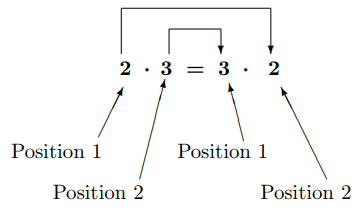$$\newcommand{\id}{\mathrm{id}}$$ $$\newcommand{\Span}{\mathrm{span}}$$ $$\newcommand{\kernel}{\mathrm{null}\,}$$ $$\newcommand{\range}{\mathrm{range}\,}$$ $$\newcommand{\RealPart}{\mathrm{Re}}$$ $$\newcommand{\ImaginaryPart}{\mathrm{Im}}$$ $$\newcommand{\Argument}{\mathrm{Arg}}$$ $$\newcommand{\norm}{\| #1 \|}$$ $$\newcommand{\inner}{\langle #1, #2 \rangle}$$ $$\newcommand{\Span}{\mathrm{span}}$$

# 2.2: The Commutative Property of Addition and Multiplication

•• Contributed by David Guichard
• Professor (Mathematics) at Whitman College
$$\newcommand{\vecs}{\overset { \rightharpoonup} {\mathbf{#1}} }$$ $$\newcommand{\vecd}{\overset{-\!-\!\rightharpoonup}{\vphantom{a}\smash {#1}}}$$$$\newcommand{\id}{\mathrm{id}}$$ $$\newcommand{\Span}{\mathrm{span}}$$ $$\newcommand{\kernel}{\mathrm{null}\,}$$ $$\newcommand{\range}{\mathrm{range}\,}$$ $$\newcommand{\RealPart}{\mathrm{Re}}$$ $$\newcommand{\ImaginaryPart}{\mathrm{Im}}$$ $$\newcommand{\Argument}{\mathrm{Arg}}$$ $$\newcommand{\norm}{\| #1 \|}$$ $$\newcommand{\inner}{\langle #1, #2 \rangle}$$ $$\newcommand{\Span}{\mathrm{span}}$$ $$\newcommand{\id}{\mathrm{id}}$$ $$\newcommand{\Span}{\mathrm{span}}$$ $$\newcommand{\kernel}{\mathrm{null}\,}$$ $$\newcommand{\range}{\mathrm{range}\,}$$ $$\newcommand{\RealPart}{\mathrm{Re}}$$ $$\newcommand{\ImaginaryPart}{\mathrm{Im}}$$ $$\newcommand{\Argument}{\mathrm{Arg}}$$ $$\newcommand{\norm}{\| #1 \|}$$ $$\newcommand{\inner}{\langle #1, #2 \rangle}$$ $$\newcommand{\Span}{\mathrm{span}}$$Figure 2.2.1

$$2$$ commuted (traveled) from position 1 to position 2

and

$$3$$ commuted (traveled) from position 2 to position 1.

Note

A commuter is a traveler. Do not say “communitive" property.

Figure 2.2.1 illustrates the commutative property of addition.

In general

$\boxed{\Large a+b=b+a}$

where $$a$$ and $$b$$ are any real numbers (like $$-6.4$$, $$\displaystyle \frac{2}{7}$$, $$\pi$$). Any real number can hide in the $$a$$-box or the $$b$$-box.

Example $$\PageIndex{1}$$: Is subtraction commutative?

Is $$3-1=1-3$$ a true statement?

Solution

No, because $$3-1-2$$ and $$1-3=-2$$. If we find one counterexample, one example that shows that subtraction is not commutative, the general property (using $$a$$ and $$b$$) does not exist.Figure 2.2.2

$$2$$ commuted (traveled) from position 1 to position 2

and

$$3$$ commuted (traveled) from position 2 to position 1.

Figure 2.2.2 illustrates the commutative property of multiplication.

In general

$\boxed{\Large ab=ba}$

where $$a$$ and $$b$$ are any real numbers (like $$-6.4$$, $$\displaystyle \frac{2}{7}$$, $$\pi$$). Any real number can hide in the $$a$$-box or the $$b$$-box.

Note that $$ab$$ means $$a$$ times $$b$$.

Example $$\PageIndex{2}$$: Is division commutative?

Is $$4\div 2=2\div 4$$ a true statement?

Solution

No, because $$4\div 2=2$$ and $$2\div 4=\displaystyle \frac{1}{2}=0.5$$. If we find one counterexample, one example that shows that division is not commutative, the general property (using $$a$$ and $$b$$) does not exist